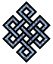fail2ban bad ip database: ip 197.248.4.24

| ip database | live view | stats | report | help | api key:

 ip: 197.248.4.24 hostname: 197.248.4.24 country:[KE] Kenya first reported: 21.11.2018 05:08.25 GMT+0200 last reported: 20.01.2019 11:58.18 GMT+0200 time period: 60d 06h 49m 53s total reports: 12 reported by: 3 host(s) filter(s): ssh (7) ssh (5) tor exit node no badips.com db Lookupport scan of '197.248.4.24':

[-hide]
```# Nmap 6.40 scan initiated Wed Nov 21 05:09:01 2018 as: /usr/bin/nmap -sU -sS -O 197.248.4.24
Nmap scan report for 197.248.4.24
Host is up (0.18s latency).
Not shown: 1000 open|filtered ports, 992 filtered ports
PORT     STATE  SERVICE
20/tcp   closed ftp-data
21/tcp   closed ftp
22/tcp   open   ssh
25/tcp   open   smtp
80/tcp   closed http
443/tcp  closed https
1433/tcp closed ms-sql-s
3389/tcp closed ms-wbt-server
No exact OS matches for host (If you know what OS is running on it, see http://nmap.org/submit/ ).
TCP/IP fingerprint:
OS:SCAN(V=6.40%E=4%D=11/21%OT=22%CT=20%CU=%PV=N%G=Y%TM=5BF4DA99%P=x86_64-pc
OS:-linux-gnu)SEQ(SP=FA%GCD=1%ISR=104%TI=Z%TS=A)OPS(O1=M564ST11NW7%O2=M564S
OS:T11NW7%O3=M564NNT11NW7%O4=M564ST11NW7%O5=M564ST11NW7%O6=M564ST11)WIN(W1=
OS:3890%W2=3890%W3=3890%W4=3890%W5=3890%W6=3890)ECN(R=Y%DF=Y%TG=40%W=3908%O
OS:=M564NNSNW7%CC=Y%Q=)T1(R=Y%DF=Y%TG=40%S=O%A=S+%F=AS%RD=0%Q=)T2(R=N)T3(R=
OS:N)T4(R=N)T5(R=Y%DF=Y%TG=40%W=0%S=O%A=S+%F=AR%O=%RD=0%Q=)T6(R=N)T7(R=N)U1
OS:(R=N)IE(R=N)

OS detection performed. Please report any incorrect results at http://nmap.org/submit/ .
# Nmap done at Wed Nov 21 05:10:01 2018 -- 1 IP address (1 host up) scanned in 60.34 seconds
```
```Σ = 38 | Δt = 0.0045571327209473s
```